# -1 - (-2). 4. a. / For each of these four expressions, -2 + 6, -(2...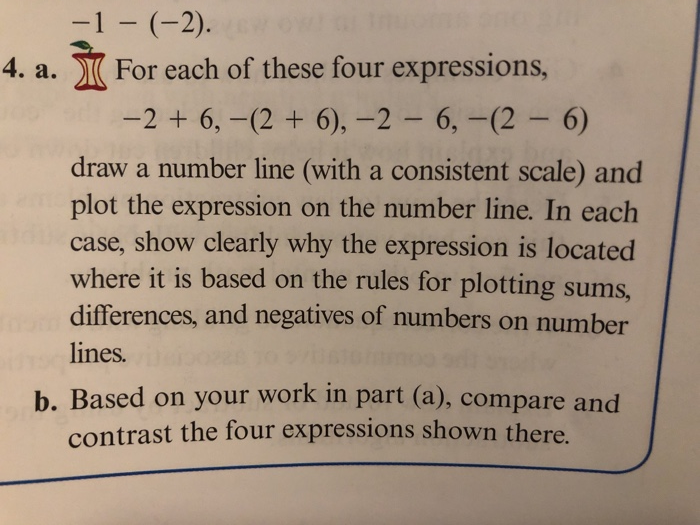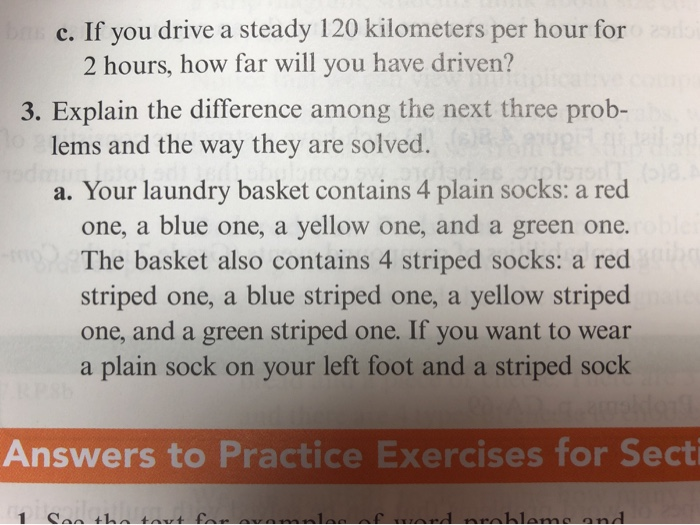-1 - (-2). 4. a. / For each of these four expressions, -2 + 6, -(2 + 6), -2 - 6,-(2 - 6) draw a number line (with a consistent scale) and plot the expression on the number line. In each case, show clearly why the expression is located where it is based on the rules for plotting sums, differences, and negatives of numbers on number lines. b. Based on your work in part (a), compare and contrast the four expressions shown there.
c. If you drive a steady 120 kilometers per hour for 2 hours, how far will you have driven? 3. Explain the difference among the next three prob- lems and the way they are solved. a. Your laundry basket contains 4 plain socks: a red one, a blue one, a yellow one, and a green one. The basket also contains 4 striped socks: a red striped one, a blue striped one, a yellow striped one, and a green striped one. If you want to wear a plain sock on your left foot and a striped sock Answers to Practice Exercises for Secti 1 Can the tant fan avomninf a nroblems and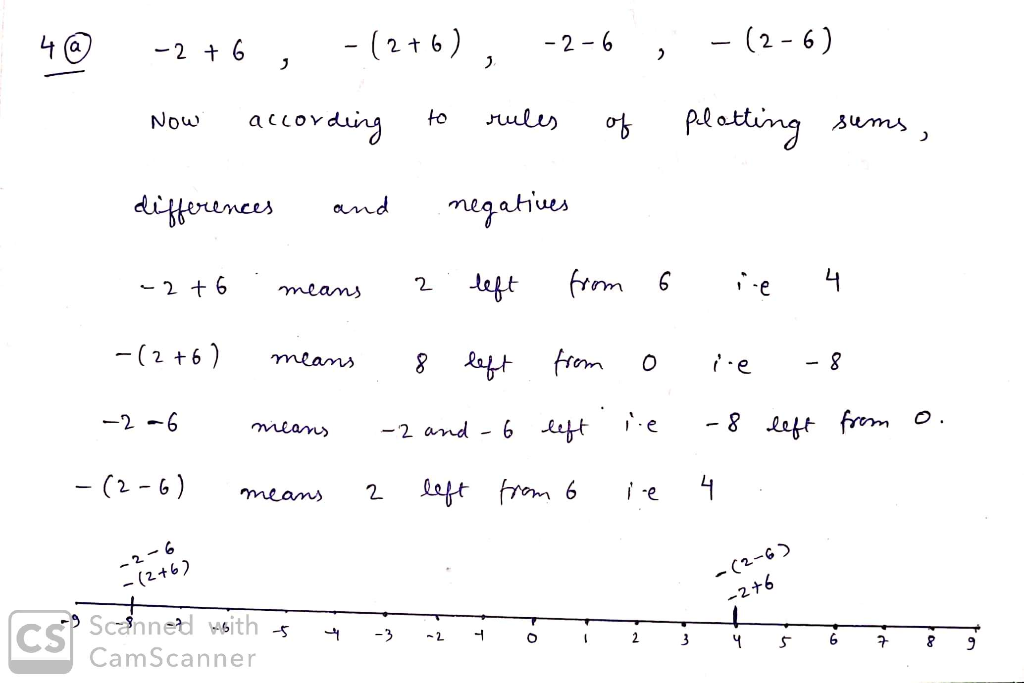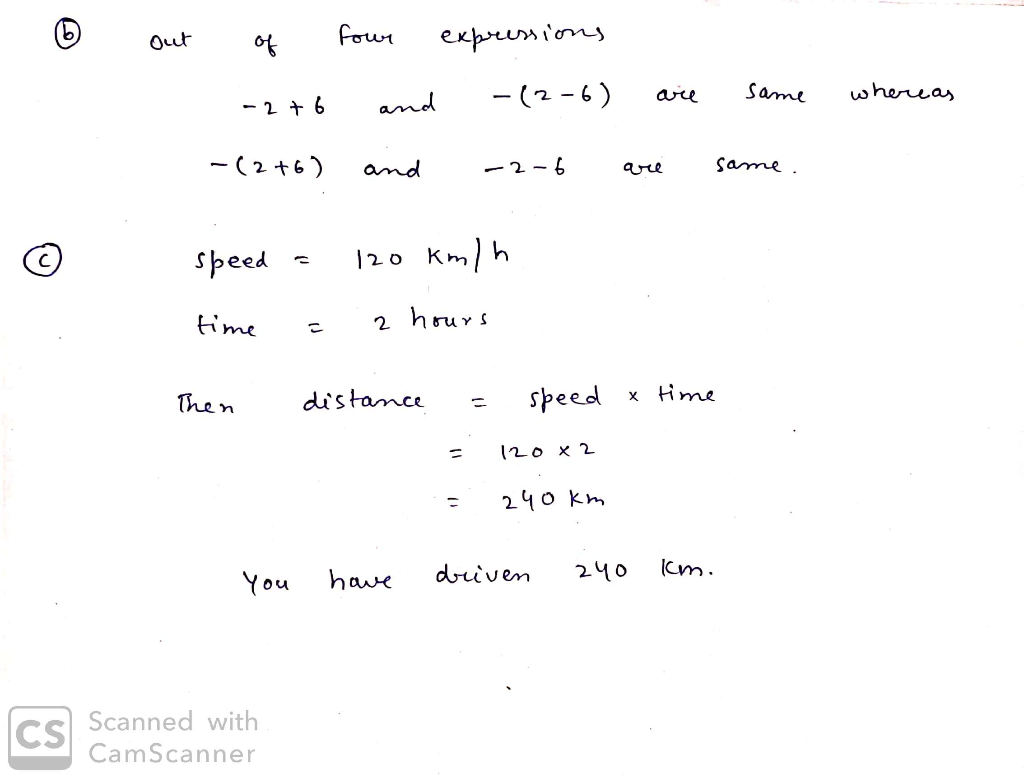#### Earn Coin

Coins can be redeemed for fabulous gifts.

Similar Homework Help Questions
• ### a drawer contains 2 red socks, 5 white socks, and 6 blue socks

a drawer contains 2 red socks, 5 white socks, and 6 blue socks. without looking, you select a sock at random, replace it, and selecct a second sock at random. what is the probability that hte first sock is blue and the second sock is red?

• ### suppos a drawer contains 6 black socks, 4 brown socks, and 2 green socks

suppos a drawer contains 6 black socks, 4 brown socks, and 2 green socks. suppose one sock gets pulled out of the drawer and that is equally likely that any one of the socks is drawn. what is the probability that the sock is either balck or green.the sock is not black.

• ### Question 3 6 pts A hat contains four red cards numbered 1 through 4; five blue...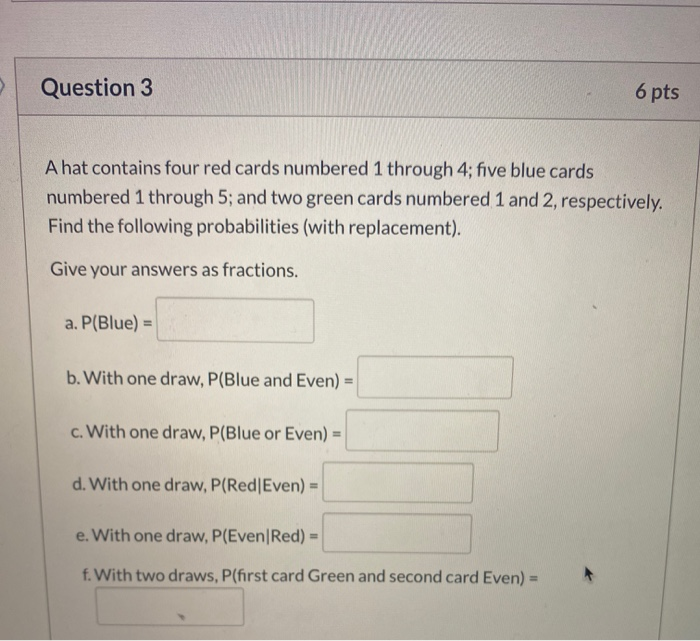Question 3 6 pts A hat contains four red cards numbered 1 through 4; five blue cards numbered 1 through 5; and two green cards numbered 1 and 2, respectively. Find the following probabilities (with replacement). Give your answers as fractions. a. P(Blue) = b. With one draw, P(Blue and Even) = c. With one draw, P(Blue or Even) = d. With one draw, P(Red Even) = e. With one draw, P(Even/Red) = f. With two draws, P(first card Green...

• ### QUESTION 2 Boolean or "truth-valued" expressions are how we express conditions that control choices and repetition...

QUESTION 2 Boolean or "truth-valued" expressions are how we express conditions that control choices and repetition in computer languages. Consider the following Python Boolean expression, where variables alpha, beta, and gamma are of type Boolean: alpha and (beta or gamma) In any algebraic notation there are usually several different ways of writing the same expression. For instance, in integer arithmetic the value of expression '4 x (5 + 2)' is numerically equivalent to that of expression '(4 x 5) +...

• ### 4. A jar contains 6 red, 5 green, 4 blue, and 2 yellow marbles. Four marbles...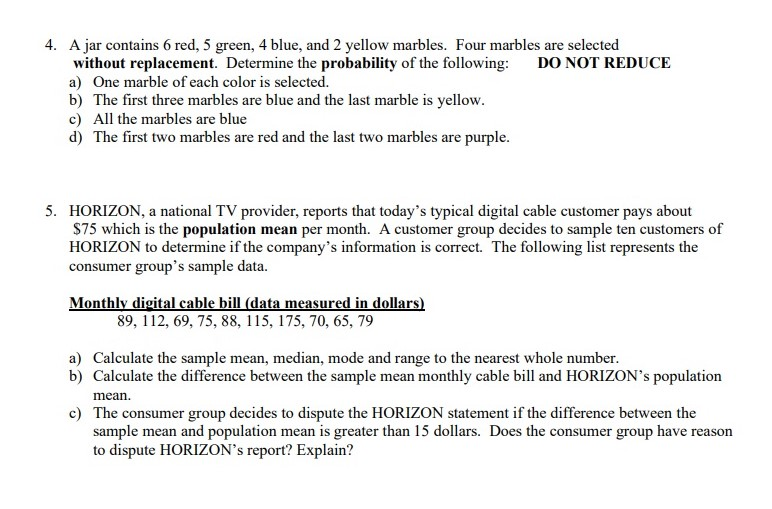4. A jar contains 6 red, 5 green, 4 blue, and 2 yellow marbles. Four marbles are selected without replacement. Determine the probability of the following: DO NOT REDUCE a) One marble of each color is selected. b) The first three marbles are blue and the last marble is yellow. c) All the marbles are blue d) The first two marbles are red and the last two marbles are purple. 5. HORIZON, a national TV provider, reports that today's typical...

• ### 4. A bag contains 1 red, 3 green, and 5 yellow balls. A sample of four...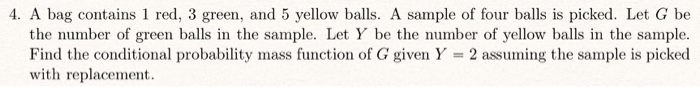4. A bag contains 1 red, 3 green, and 5 yellow balls. A sample of four balls is picked. Let G be the number of green balls in the sample. Let Y be the number of yellow balls in the sample. Find the conditional probability mass function of G given Y = 2 assuming the sample is picked with replacement.

• ### 4. A bag contains 1 red, 3 green, and 5 yellow balls. A sample of four...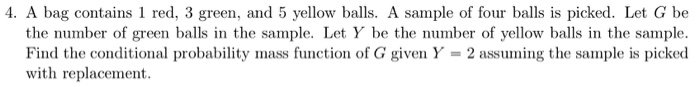4. A bag contains 1 red, 3 green, and 5 yellow balls. A sample of four balls is picked. Let G be the number of green balls in the sample. Let Y be the number of yellow balls in the sample. Find the conditional probability mass function of G given Y 2 assuming the sample is picked with replacement

• ### You mix the letters S, E, M, I, T, R, O, P, I, C, A, and L thoroughly

1. You mix the letters S, E, M, I, T, R, O, P, I, C, A, and L thoroughly. Without looking, you draw one letter. Find the probability that you select a vowel. Write your answer as a fraction in simplest form. (1 point)2. Suppose you spin the spinner once. Find the probability.P(green) (1 point)3. Suppose you spin the spinner once. Find the probability.P(yellow or green) (1 point)4. You mix the letters S, E, L, E, C, T, E, and...

• ### Four balls are to be randomly chosen from an urn containing 4 red, 5 green, and...

Four balls are to be randomly chosen from an urn containing 4 red, 5 green, and 6 blue balls. 1. Find the probability that at least one red ball is chosen? 2. Given that no red balls are chosen, what is the probability that there are exactly 2 green balls among the four balls chosen.

• ### 2) A deck of 24 cards contains six blue cards numbered 1 through 6, six red...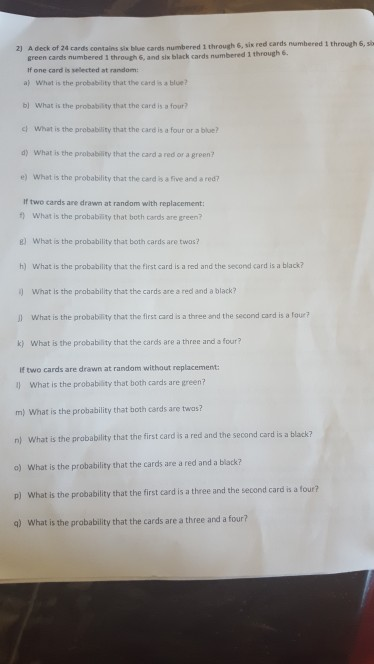2) A deck of 24 cards contains six blue cards numbered 1 through 6, six red cards numbered 1 through 6, s hrough 6. green cards numbered 1 through 6, and six black cards numbered 1 t If one card is selected at random: a) What i, the probability that the card is a ???? b) What is the probability that the card is a four? c) What is the probability that the card is a four or a blue?...